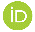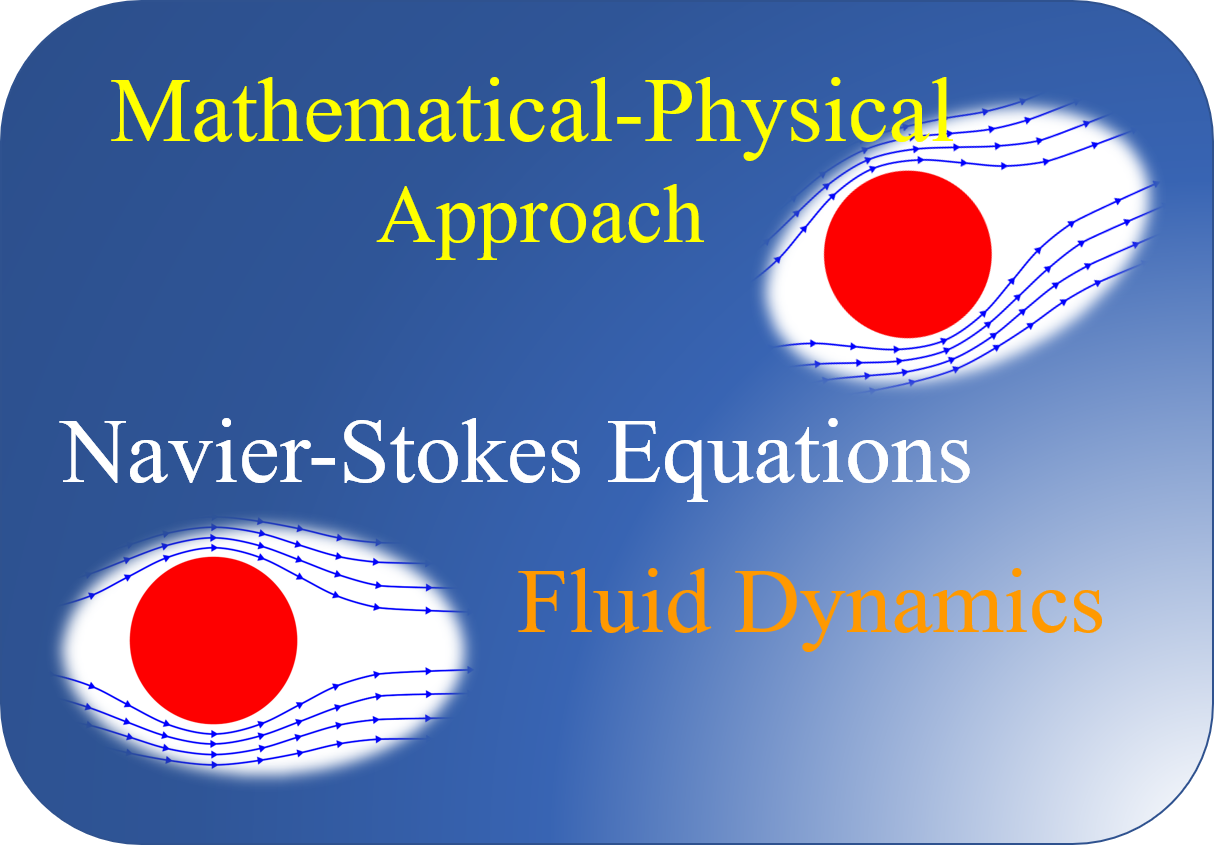# Mathematical-Physical Approach to Prove that the Navier-‎Stokes Equations Provide a Correct Description of Fluid ‎Dynamics

## Authors

• Andreas Martin Postfach (PO Box ) 2102, 26655 Westerstede, Germany‎

## Keywords:

Navier-Stokes equations, Fluid dynamics

## Abstract

This publication takes a mathematical approach to a general solution to the Navier-Stokes equations. The basic idea is a mathematical analysis of the unipolar induction according to Faraday with the help of the vector analysis. The vector analysis enables the unipolar induction and the Navier-Stokes equations to be related physically and mathematically, since both formulations are mathematically equivalent. Since the unipolar induction has proven itself in practice, it can be used as a reference for describing the Navier-Stokes equations.

## Author Biography

### Andreas Martin, Postfach (PO Box ) 2102, 26655 Westerstede, Germany‎

Andreas MartinPostfach (PO Box ) 2102, 26655 Westerstede, Germany

## References

‎‎ A. G. J. R. o. M. P. Ramm, "Navier-Stokes ‎equations paradox," vol. 88, no. 1, pp. 41-45, 2021.‎

‎‎ H. Grassmann, Die lineale Ausdehnungslehre ein ‎neuer Zweig der Mathematik: dargestellt und durch ‎Anwendungen auf die übrigen Zweige der ‎Mathematik, wie auch auf die Statik, Mechanik, die ‎Lehre vom Magnetismus und die Krystallonomie ‎erläutert. O. Wigand, 1844.‎

‎‎ R. Becker, Theorie der Elektrizität: Band 1: ‎Einführung in die Maxwellsche Theorie, ‎Elektronentheorie. Relativitätstheorie. Springer-‎Verlag, 2013.‎

‎‎ A. Quarteroni, "Reduced basis approximation for ‎parametrized partial differential equations," in ‎Numerical Models for Differential Problems: ‎Springer, 2014, pp. 585-633.‎

‎‎ T. Schneider, "Die Unipolarmaschine zweiter Art," ‎‎2015.‎

‎‎ F. Irgens, Continuum mechanics. Springer Science & ‎Business Media, 2008.‎

‎‎ L. Barreiro, J. Campanha, R. J. P. A. S. M. Lagos, and ‎i. Applications, "The thermohydrodynamical picture ‎of Brownian motion via a generalized Smoluchowsky ‎equation," vol. 283, no. 1-2, pp. 160-165, 2000.‎2022-09-03

## How to Cite

Martin, A. (2022). Mathematical-Physical Approach to Prove that the Navier-‎Stokes Equations Provide a Correct Description of Fluid ‎Dynamics: Mathematical Physics. Hyperscience International Journal, 2(3), 97–102. https://doi.org/10.55672/hij2022pp97-102

## Section

Physic